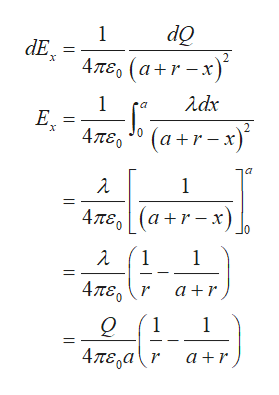Positive charge Q is distributed uniformly along the x-axis from x=0 to x=a. A positive point charge q is located on the positive x-axis at x=a+r, a distance r to the right of the end of Q.Calculate the x-component of the electric field produced by the charge distribution Q at points on the positive x-axis where x>a.Express your answer in terms of the variables Q, a, r, and and appropriate constants. Calculate the magnitude of the force that the charge distribution Q exerts on q.Express your answer in terms of the variables Q, q, a, r, and appropriate constants. Calculate the direction of the force that the charge distribution Q exerts on q.

Question

Positive charge Q is distributed uniformly along the x-axis from x=0 to x=a. A positive point charge q is located on the positive x-axis at x=a+r, a distance r to the right of the end of Q.

Calculate the x-component of the electric field produced by the charge distribution Q at points on the positive x-axis where x>a.
Express your answer in terms of the variables Q, a, r, and and appropriate constants.

Calculate the magnitude of the force that the charge distribution Q exerts on q.
Express your answer in terms of the variables Q, q, a, r, and appropriate constants.

Calculate the direction of the force that the charge distribution Q exerts on q.
Step 1

The charge Q is uniformly distributed along the axis x = 0 to x = a. The linear charge density of the charge distribution is

Step 2

The charge across the small segment is

Step 3

The electric field strength due...help_outlineImage Transcriptionclose1 dE Απε, (α+r- 3)" δ Χ λάκ 1 E Е, х 0 (a+r- s) 2 4πεο λ 1 4πε (a+r- ) λ 1 1 4πε, r α+r 1 1 4πε,α (r α+r fullscreen

Want to see the full answer?

See Solution

Want to see this answer and more?

Our solutions are written by experts, many with advanced degrees, and available 24/7

See Solution
Tagged in

Science